Courses

# Hydrostatic Forces On Surfaces And Fluid Kinematics - MCQ Test 2

## 30 Questions MCQ Test Mock Test Series - Mechanical Engineering (ME) for GATE 2020 | Hydrostatic Forces On Surfaces And Fluid Kinematics - MCQ Test 2

Description
This mock test of Hydrostatic Forces On Surfaces And Fluid Kinematics - MCQ Test 2 for Mechanical Engineering helps you for every Mechanical Engineering entrance exam. This contains 30 Multiple Choice Questions for Mechanical Engineering Hydrostatic Forces On Surfaces And Fluid Kinematics - MCQ Test 2 (mcq) to study with solutions a complete question bank. The solved questions answers in this Hydrostatic Forces On Surfaces And Fluid Kinematics - MCQ Test 2 quiz give you a good mix of easy questions and tough questions. Mechanical Engineering students definitely take this Hydrostatic Forces On Surfaces And Fluid Kinematics - MCQ Test 2 exercise for a better result in the exam. You can find other Hydrostatic Forces On Surfaces And Fluid Kinematics - MCQ Test 2 extra questions, long questions & short questions for Mechanical Engineering on EduRev as well by searching above.
QUESTION: 1

Solution:

Ans. (b)

QUESTION: 2

Solution:

Ans. (c)

QUESTION: 3

### Existence of velocity potential implies that

Solution:

Ans. (b)

QUESTION: 4

In a flow field, the streamlines and equipotential lines

Solution:

Ans. (d)

QUESTION: 5

Assertion (A): The local acceleration is zero in a steady motion.

Reason (R): The convective component arises due to the fact thata fluid element experiences different velocities at differentlocations.

Solution:

Ans. (b) • A flow is said to be steady when conditions do not change with time at any point.
• In a converging steady flow, there is only convective acceleration.
• Local acceleration is zero in steady flow.

QUESTION: 6

Match List-I (Flows Over or Inside the Systems) with List-II (Type of Flow) and select the correct answer: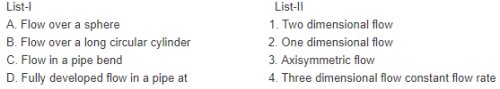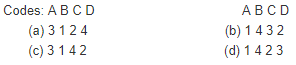Solution:

Ans. (c)

QUESTION: 7

Which one of the following statements is correct?
Irrotational flow is characterized as the one in which

Solution:

Ans. (c)

QUESTION: 8

Match List-I with List-II and select the correct answer using the code given below the lists: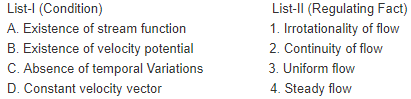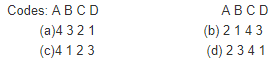Solution:

Ans. (b)

QUESTION: 9

A streamline is a line:

Solution:

Ans. (d)

QUESTION: 10

Assertion (A): Streamlines can cross one another if the fluid hashigher velocity.
Reason (R): At sufficiently high velocity, the Reynolds number ishigh and at sufficiently high Reynolds numbers, the structure ofthe flow is turbulent type.

Solution:

Ans. (d)

QUESTION: 11

If for a flow, a stream function

• exists and satisfies the Laplaceequation, then which one of the following is the correctstatement?
Solution:

Ans (a) if a stream function

• exists means a possible case of flow, if it satisfies the Laplace equation then flow is irrotational.
QUESTION: 12

The velocity potential of a velocity field is given by = x2 – y2 +const. Its stream function will be given by:

Solution:

Ans. (b) Use Cauchy – Riemann equation

QUESTION: 13

Which one of the following statements is true to two dimensional flow of ideal fluids?

Solution:

Ans. (d) For a possible case of fluid flow Stream function will exist, but potential function will exist only for irrotational flow. In this case flow may be rotational or irrotational.

QUESTION: 14

What is the vertical component of pressure force on submerged curved surface equal to?

Solution:

Ans. (d) The vertical component of the hydrostatic force on a submerged curved surface is the weight of the liquid vertically above it.

QUESTION: 15

A plate of rectangular shape having the dimensions of 0.4m x0.6m is immersed in water with its longer side vertical. The totalhydrostatic thrust on one side of the plate is estimated as 18.3kN. All other conditions remaining the same, the plate is turnedthrough 90o such that its longer side remains vertical. What would be the total force on one of the plate?

Solution:

Ans. (b)

QUESTION: 16

What is the vertical component of pressure force on submergedcurved surface equal to?

Solution:

Ans. (d) The vertical component of the hydrostatic force on a submerged curved surface is the weight of the liquid vertically above it.

QUESTION: 17

A rectangular tank of base 3 m × 3 m contains oil of specificgravity 0.8 upto a height of 8 m. When it is accelerated at 2.45m/s2 vertically upwards, the force on the base of the tank will be:

Solution:

Ans. (c)

QUESTION: 18

A vertical dock gate 2 meter wide remains in position due to horizontal force of water on one side. The gate weights 800 Kg and just starts sliding down when the depth of water upto the bottom of the gate decreases to 4 meters. Then the coefficient of friction between dock gate and dock wall will be:

Solution:

Ans. (c)
μP =W
or
μρg(4´ 2).(4 / 2) = 800 x g
or
μ = 0.05

QUESTION: 19

A circular plate 1.5 m diameter is submerged in water with its greatest and least depths below the surface being 2 m and 0.75m respectively. What is the total pressure (approximately) onone face of the plate?

Solution:

Ans. (c)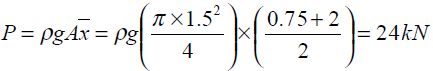QUESTION: 20

The hydrostatic force on the curvedsurface AB shown in given figure acts.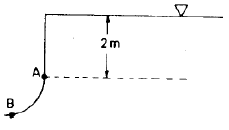Solution:

Ans. (d) Total hydrostatic force on the curved surface is upwards, but at an angle with the vertical plane.

QUESTION: 21

Assertion (A): A circular plate is immersed in a liquid with its periphery touching the free surface and the plane makes anangle q with the free surface with different values of q , the position of centre of pressure will be different.
Reason (R): Since the center of pressure is dependent on second moment of area, with different values of q , second moment ofarea for the circular plate will change.

Solution:

Ans. (c)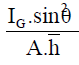QUESTION: 22

A circular annular plate bounded by two concentric circles of diameter 1.2m and 0.8 m is immersed in water with its plane making an angle of 45o with the horizontal. The centre of the circles is 1.625m below the free surface. What will be the total pressure force on the face of the plate?

Solution:

Ans. (b)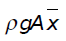=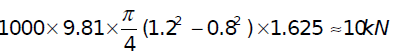QUESTION: 23

A circular plate 1.5 m diameter is submerged in water with its greatest and least depths below the surface being 2 m and 0.75m respectively. What is the total pressure (approximately) onone face of the plate?

Solution:

Ans. (c)

QUESTION: 24

The realisation of velocity potential in fluid flow indicates thatthe

Solution:

Ans. (a) The realisation of velocity potential in fluid flow indicates that the flow must be irrotational.

QUESTION: 25

A cylindrical vessel having its height equal to its diameter is filled with liquid and moved horizontally at acceleration equal to acceleration due to gravity. The ratio of the liquid left in the vessel to the liquid at static equilibrium condition is:

Solution:

Ans. (c)

QUESTION: 26

Consider the following statements:
1. Streak line indicates instantaneous position of particles offluid passing through a point.
2. Streamlines are paths traced by a fluid particle with constantvelocity.
3. Fluid particles cannot cross streamlines irrespective of thetype of flow.
4. Streamlines converge as the fluid is accelerated, and diverge when retarded.
Which of these statements are correct?

Solution:

Ans. (b) 2 is wrong.

QUESTION: 27

Which one of the following statements is correct?  ]A steady flow of diverging straight stream lines

Solution:

Ans. (c)

QUESTION: 28

In a steady, incompressible, two dimensional flow, one velocity component in the x-direction is given by u = cx2/y2. The velocity component in the y-direction will be:

Solution:

Ans. (b)

QUESTION: 29

A liquid mass readjusts itself and undergoes a rigid body type ofmotion when it is subjected to a

Solution:

Ans. (a)

QUESTION: 30

For a stream function to exist, which of the following conditions should hold?
1. The flow should always be irrotational.
2. Equation of continuity should be satisfied.
3. The fluid should be incompressible.
4. Equation of continuity and momentum should be satisfied.Select the correct answer using the codes given below:
Codes:

Solution:

Ans. (d)